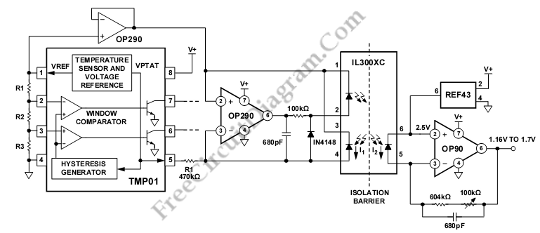# IL300XC Isolation Amplifier for TMP01 Temperature SensorThis schematic diagram is about IL300XC Isolation Amplifier circuit for TMP01 Temperature Sensor. This circuit is used in an environment that needs to be electrically isolated from the central processing area. This circuit uses an 8-pin optoisolator (IL300XC). IL300XC was chosen because it can operate across a 5,000V. To drive the LED connected between Pin 2 and Pin 1, this circuit uses an OP290 single-supply amplifier. the photodiode connected from Pin 3 to Pin 4 gives the feedback. Here is the schematic diagram of the  circuit:The OP290 drives the LED, that there is enough current generated in the photodiode to exactly equal the current derived from the VPTAT voltage across the 470 kO resistor. On the receiving end, the current from the second photodiode is converted to a voltage through its feedback resistor R2.

TO buffer the 2.5 V reference voltage of the TMP01, this circuit uses the other amplifier in the dual OP290. It will give the an accurate, low drift LED bias level without affecting the programmed hysteresis current. The bias level accuracy at receiving end is provided by A REF43.

The current of the photodiode is determined by following equation:
I1=(2.5V-VPTAT)/470K
The output voltage is determined by following equation:

Vout=2.5V-I2*R2
=2.5V-0.7*((2,5V-VPTAT)/470)*644K=VPTATT

R2 must be larger than R1 to achieve overall unity gain because the gain of IL300XC is less than 1.0. To correct for the initial gain accuracy of the IL300XC, A trim is used in this circuit. Just adjust the trim to get output voltage equal to VPTAT at any particular temperature.

Both the OP90 and REF43 contribute no significant error because of drift and operate from a single supply. [Circuit’s schematic diagram source: analog.com]